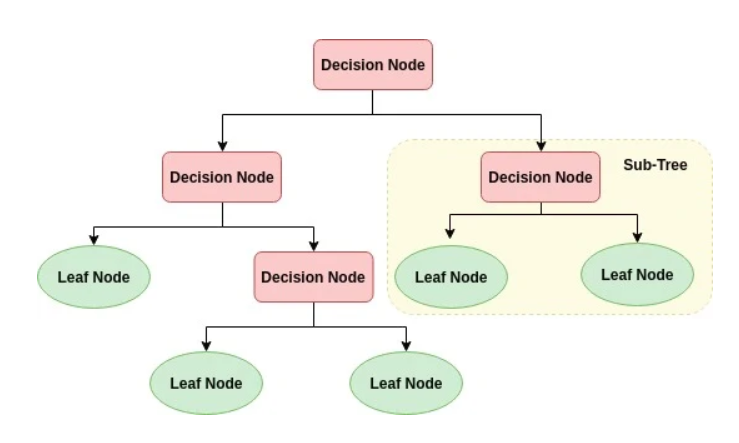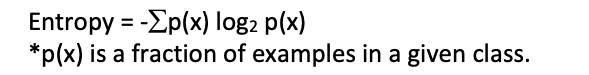top of pageSearch

# Decision Tree, Information Gain and Gini Index for Dummies

Decision Tree can be defined as a diagram or a chart that people use to determine a course of action or show a statistical probability. It represents a possible decision, outcome or reaction and an end result.

A Decision Tree is a structure that includes decision nodes, branches, and leaf nodes. Each internal node signifies a test on an attribute, each branch denotes the outcome of a test, and each leaf node holds a class label.Decision Trees are used to find an answer to a complex problem.

The following methods could be used to achieve these answers:

1. Information Gain

2. Gini Index

3. Gain Ratio

Let us further understand how to calculate Information Gain and Gini Index with the help of an example.

Insomnia is a sleep disorder marked by problems getting to sleep, staying asleep and sleeping as long as one would like. It can have serious effects, leading to excessive lethargy, a higher risk of accidents and health effects from sleep deprivation.

A few major causes of Insomnia are irregular sleep schedule, unhealthy eating habits, illness and pain, bad lifestyle and stress. The following is the data we will use:Information Gain - It is the main key that is used by decision tree Algorithms to construct it. It measures how much information a feature gives us about the class.

Information Gain = entropy(parent) – [weighted average] * entropy(children)

Entropy – It is the impurity in a group of examples. Information gain is the decrease in entropy.1. Analysis of Sleep Schedule feature:

Let us consider the values of the Insomnia column as the parent node (Acute, Acute, Hyper, Hyper) and the values of Sleep Schedule column as the children node (On Track, On Track, On Track, Off Track).

P(Acute) = fraction of Acute examples in parent node = 2/4 = 0.5

P(Hyper) = fraction of Hyper examples in parent node = 2/4 = 0.5

Therefore, Entropy of Parent Node:= - [0.5 log2 (0.5) + 0.5 log2 (0.5)]

= - [- 0.5 + (- 0.5)]

= 1

Entropy of left child node (On Track) = Acute, Acute, Hyper = 0.9

Entropy of right child node (Off Track) = Hyper = 0[Weighted Average] Entropy (children) = 3/4 * 0.9 + 1/4 * 0

= 0.675

Information Gain of Sleep Schedule

= entropy(parent) – [weighted average] * entropy(children)

= 1 – 0.675

Information Gain = 0.325

2. Analysis of Eating Habits feature:

Entropy of left child node (Healthy) is Acute, Hyper

Entropy of right child node (Unhealthy) is Acute, Hyper

Information Gain = Entropy(parent) – [Weighted average] * Entropy (children)

= 1 - (2/4 * 1 + 2/4 * 1)

= 1 - 1

Information Gain = 0

3. Analysis of Lifestyle feature:

Entropy of left child node of (Good) = Acute, Acute

Entropy of right child node of (Bad) = Hyper, Hyper

Information Gain = Entropy(parent) – [Weighted average] * Entropy (children)

= 1 - (2/4 * 0 + 2/4 * O)

= 1 - 0

Information Gain = 1

4. Analysis of Stress feature:

Entropy of left child node of (Stressed) = Acute, Hyper

Entropy of right child node of (Relaxed) = Acute, Hyper

Information Gain = Entropy(parent) – [Weighted average] * Entropy (children)

= 1 - (2/4 * 1 + 2/4 * 1)

= 1 - 1

Information Gain = 0

As per the calculations above, the information gain of Sleep Schedule is 0.325, Eating Habits is 0, Lifestyle is 1 and Stress is 0. So, the Decision Tree Algorithm will construct a decision tree based on feature that has the highest information gain. In our case it is Lifestyle, wherein the information gain is 1.

Gini Index - Gini Index or Gini Impurity is the measurement of probability of a variable being classified wrongly when it is randomly chosen. The degree of Gini Index varies from zero to one.

Formula –Here, (Pi) is the probability of an element classified wrongly.

1. Analysis of Sleep Schedule feature:

P (On Track) = 3/4

P (Off Track) = 1/4

Sleep Schedule = On Track and Insomnia = Acute = 2/3

Sleep Schedule = On Track and Insomnia = Hyper = 1/3

Gini Index (On Track) = 1 - [(2/3)2 + (1/3)2]

= 1 - [ 0.44 + 0.11]

= 0.45

Sleep Schedule = Off Track and Insomnia = Acute = 0

Sleep Schedule = Off Track and Insomnia = Hyper = 1

Gini Index (Off Track) = 1 - [02 + 12]

= 0

Weighted sum of Gini indices (Sleep Schedule)

= [3/4 * 0.45] + [1/4 * 0]

= 0.3375

2. Analysis of Eating Habits feature:

P (Healthy) = 2/4

P (Unhealthy) = 2/4

Eating Habits = Healthy and Insomnia = Acute = 1/2

Eating Habits = Healthy and Insomnia = Hyper = 1/2

Gini Index (Healthy) = 1 - [(1/2)2 + (1/2)2]

= 0.5

If (Eating Habits = Unhealthy and Insomnia = Acute): 1/2

If (Eating Habits = Unhealthy and Insomnia = Hyper): 1/2

Gini Index (Unhealthy) = 1 - [(1/2)2 + (1/2)2]

= 0.5

Weighted sum of Gini indices (Eating Habits)

= [2/4 *0.5] + [2/4*0.5]

= 0.25 + 0.25

= 0.5

3. Analysis of Lifestyle feature:

P(Good) = 2/4

Lifestyle = Good and Insomnia = Acute = 1

Lifestyle = Good and Insomnia = Hyper = 0

Gini Index (Good) = 1 - [12 + 0]

= 0

Lifestyle = Bad and Insomnia = Hyper = 0

Lifestyle = Bad and Insomnia = Hyper = 1

Gini Index(Bad) = 1 - [02 + 12]

= 0

Weighted sum of Gini indices (Lifestyle)

= [2/4 *0] + [2/4 * 0]

= 0

4. Analysis of Stress feature:

P (Stressed) = 2/4

P (Relaxed) = 2/4

Stress = Stressed and Insomnia = Acute = ½

Stress = Stressed and Insomnia = Hyper = ½

Gini Index (Stressed) = 1 - [(1/2)2 + (1/2)2] = 0.5

Stress = Relaxed and Insomnia = Acute = 1/2

Stress = Relaxed and Insomnia = Hyper = 1/2

Gini Index (Relaxed) = 1 - [(1/2)2 + (1/2)2] = 0.5

Weighted sum of Gini indices (Stress)

= [2/4 *0.5] + [2/4*0.5]

= 0.25 + 0.25

= 0.5

Therefore,

Gini index for Sleep Schedule is 0.3375

Gini index for Eating Habits is 0.5

Gini index for Lifestyle is 0

Gini index for Stress is 0.5

Since we have the Gini Index of Lifestyle to be 0, it denotes that it is pure and insomnia majorly depends upon the kind of Lifestyle a patient is leading.

Unhealthy habits and routines related to lifestyle and food and drink can increase a person’s risk of insomnia. Habits like working late, uncontrolled screen time, a long afternoon nap, excessive caffeine and alcohol consumption are a few lifestyle factors can cause wakefulness.

There are many other factors like illness, pain, mental health disorders, anxiety, depression, side effects of medication, neurological problems, specific sleep disorders like restless leg syndrome.

Using Decision Tree to process data available to find out the highest determining cause of insomnia is the best choice. Decision Trees are very simple to understand because of their visual representation. They can handle a large quantity of quality data which can be validated using statistical sets and are computationally inexpensive. They are a white box type of Machine Learning algorithm as compared to the Neural Network black box. It is also a distribution free method which does not depend upon probability distribution assumptions and lastly because it can handle high dimensional data with really good accuracy.